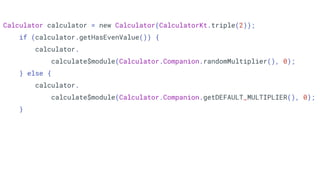Ce diaporama a bien été signalé.
Le téléchargement de votre SlideShare est en cours. ×

# Javantura v6 - Kotlin-Java Interop - Matej Vidaković

Publicité
Publicité
Publicité
Publicité
Publicité
Publicité
Publicité
Publicité
Publicité
Publicité
PublicitéChargement dans…3
×

## Consultez-les par la suite

1 sur 17 Publicité

# Javantura v6 - Kotlin-Java Interop - Matej Vidaković

While Kotlin is designed to work well with Java by default, we'll still need to some work to get clean and idiomatic code in both languages.
In this talk we'll cover both how to make your Java code more Kotlin friendly and how to make your Kotlin code nicer to use from Java.

While Kotlin is designed to work well with Java by default, we'll still need to some work to get clean and idiomatic code in both languages.
In this talk we'll cover both how to make your Java code more Kotlin friendly and how to make your Kotlin code nicer to use from Java.

Publicité
Publicité

### Javantura v6 - Kotlin-Java Interop - Matej Vidaković

1. 1. Kotlin - Java Interop Matej Vidaković
2. 2. Doesn’t it just work?
3. 3. Calculator calculator = new Calculator(CalculatorKt.triple(2)); if (calculator.getHasEvenValue()) { calculator. calculate\$module(Calculator.Companion.randomMultiplier(), 0); } else { calculator. calculate\$module(Calculator.Companion.getDEFAULT_MULTIPLIER(), 0); }
4. 4. Kotlin - Java differences ● null safety ● extension functions ● properties ● companion objects ● checked exceptions ● primitive types ● static members ● visibility modifiers
5. 5. fun Int.triple(): Int = this * 3 class Calculator(val value: Int) { val hasEvenValue: Boolean = value % 2 == 0 internal fun calculate(multiplier: Int, offset: Int = 0) = value * multiplier + offset companion object { val DEFAULT_MULTIPLIER = 1 fun randomMultiplier() = Random.nextInt() } }
6. 6. Top level functions @file:JvmName("IntUtils") @file:JvmMultifileClass package hr.matvidako CalculatorKt.triple(2) IntUtils.triple(2)
7. 7. Renaming getters @get:JvmName("hasEvenValue") val hasEvenValue: Boolean = value % 2 == 0 calculator.hasEvenValue() calculator.getHasEvenValue()
8. 8. Internal methods @JvmName("calculate") internal fun calculate(multiplier: Int, offset: Int = 0) calculator.calculate\$module(Calculator.Companion.randomMultiplier(), 0) calculator.calculate(Calculator.Companion.randomMultiplier(), 0)
9. 9. Default param values @JvmOverloads @JvmName("calculate") internal fun calculate(multiplier: Int, offset: Int = 0) calculator.calculate(Calculator.Companion.randomMultiplier(), 0) calculator.calculate(Calculator.Companion.randomMultiplier())
10. 10. Static methods @JvmStatic fun randomMultiplier() = Random.nextInt() calculator.calculate(Calculator.randomMultiplier()) calculator.calculate(Calculator.Companion.randomMultiplier())
11. 11. Exposing property as field @JvmField val DEFAULT_MULTIPLIER = 1 calculator.calculate(Calculator.DEFAULT_MULTIPLIER) calculator.calculate(Calculator.getDEFAULT_MULTIPLIER());
12. 12. Calculator calculator = new Calculator(IntUtils.triple(2)); if (calculator.hasEvenValue()) { calculator.calculate(Calculator.randomMultiplier()); } else { calculator.calculate(Calculator.DEFAULT_MULTIPLIER); }
13. 13. @file:JvmName("IntUtils") @file:JvmMultifileClass package hr.matvidako fun Int.triple(): Int = this * 3 class Calculator(val value: Int) { @get:JvmName("hasEvenValue") val hasEvenValue: Boolean = value % 2 == 0 @JvmOverloads @JvmName("calculate") internal fun calculate(multiplier: Int, offset: Int = 0) = value * multiplier + offset companion object { @JvmField val DEFAULT_MULTIPLIER = 1 @JvmStatic fun randomMultiplier() = Random.nextInt() } }
14. 14. That’s a lot of annotations…
15. 15. Kotlin friendly Java ● add Nullability annotations ● avoid using Kotlin keywords in method / field names ○ `as`, `in`, `when` ● use standard get, set method prefixes ○ allows property syntax in Kotlin ● put params eligible for SAM conversion last ○ allows use of trailing lambda syntax
16. 16. In conclusion...
17. 17. Thank you